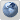DE5T1NY's
Would you like to react to this message? Create an account in a few clicks or log in to continue.

# what is Number Bonds##what is Number Bonds

What does Number Bonds mean in maths?

A number bond is a mental picture of the relationship between a number and the parts that combine to make it, often referred to as "number pairs". The concept of number bonds is very basic, an important foundation for understanding how numbers work. A whole thing is made up of parts.

To us they are simply the pairs of numbers that make up a given number.

Example:

Number bonds to 10 = 1 + 9, 2 + 8, 3 + 7, 4 + 6, 5 + 5

Number bonds to 20 = 1 + 19, 2 + 18, 3 + 17, 4 + 16, 5 + 15

Children might be given a number, such as 5, and then asked to select two groups of objects that will add up to that number.

Example:

0 + 5, 1 + 4, 2 + 3

They also need to be confident with the corresponding subtraction facts.

Example: 10 - 3 = 7
Example: 20 - 13 = 7 and so on.

Give your child ten or twenty counters and ask them questions such as: What do you add to 4 to make 10? What do you need to subtract from 20 to make 14? Encourage them to use the counters to work it out.

Different shaded counters in groups of ten or twenty might made the child more interested in doing these tasks.

Remember number bonds don't need to run in a sequence of numbers 0 to 10 when adding together.

Example: Number bonds to 8

Can be seen as 1 + 7 or 7 + 1, 2 + 6 or 6 + 2 and so on.

So for the example above there is a combined "pairing" of 9 different Number bonds to reach 8 using the 10 counter method, while using the subtraction method only requires a combined "pairing" of 3 different Number bonds ( 10 - 2, 9 - 1, 8 - 0 ).

Sounds like the subtraction method is easier than the adding method for us to teach our kids, but it's not that easy.

Children start of in life learning to count up in "ones" usually its Birthday counting like your 4 this year and next year you'll be 5 (4 + 1 = 5) slowly they learn to count to 10 then it's followed by sequences to 10 ( 20, 30, 40 and so on up to 100), but somehow the subtraction method don't seem to enter their young lives at this early stage. Think most teacher would agree that adding is easier to learn than subtraction at the child's early years at school.DE5T1NY

Posts : 244
Join date : 2018-09-09##NUMBER BONDS 10DE5T1NY